# PSEB 5th Class Maths Solutions Chapter 6 Measurement Ex 6.7

Punjab State Board PSEB 5th Class Maths Book Solutions Chapter 6 Measurement Ex 6.7 Textbook Exercise Questions and Answers.

## PSEB Solutions for Class 5 Maths Chapter 6 Measurement Ex 6.7

1. Find the difference :

Question 1.
8 hours 30 min and 2 hours 10 min
Solution: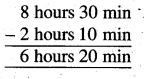Question 2.
10 hours 30 min 20 sec and 8 hours 20 min 15 sec
Solution: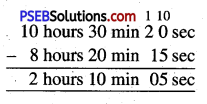Question 3.
11 years 5 months and 6 years
Solution: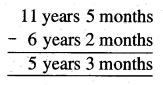Question 4.
7 years 2 months and 3 years
Solution: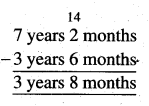Note, ∵ 1 year = 12 months
∴ 12 months + 2 months = 14 months

2. Find the Time :

Question 1.
4 hours before 5:30 pm
Solution:
4 hours before 5:30 pm
4 hours = 30 minutes + 3 hours + 30 minutes
30 minutres before 5:30 pm = 5.00 pm
3 hours before 5:00 pm = 2:00 pm
30 minutes before 2:00 pm = 1:30 pmQuestion 2.
2 hours after 11:00 am
Solution:
2 hours after 11:00 am
1 hour after 11:00 am = 12:00 noon
1 hour after 12:00 noon =1:00 pm
Second method :
2 hours after 11:00 am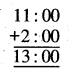i.e. 12:00 +1:00
= 1:00 pm

Question 3.
6 hours before 4:30 am
Solution:
6 hours before 4:30 am
6 hours = 30 minutes + 4 hours + 1 hour + 30 minutes
30 minutes before 4:30 am = 4:00 am
4 hours before 4:00 am = 12:00 mid night
1 hour before 12:00 mid night = 11:00 pmQuestion 4.
1 hour 45 min after 8:30 am
Solution:
1 hour 45 min after 8:30 am
1 hour 45 minutes = 30 minutes + 1 hour + 15 minutes
30 minutes after 8:30 am = 9:00 am
1 hour after 9:00 am = 10: 00 am
15 minutes after 10:00 am = 10:15 am

3. Find the Time Gap :

Question 1.
From 3:00 am to 10:00 am
Solution:
Time gap from 3:00 am to 10:00 am = 7 hourQuestion 2.
From 6:00 am to 1:30 pm
Solution:
Time gap from 6:00 am to 1:30 pm
Time gap between 6:00 am to 12:00 noon = 6 hours
Time gap from 12:00 noon to 1:00 pm = 1 hour
Time gap from 1:00 pm to 1:30 pm = 30 minutes
Hence, time gap from 6:00 am to 1:30 pm = 7 hours 30 minutes

Second Method :
1:30 pm = 1:30 + 12:00 = 13:30 o’clock
Time gap from 6:00 am to 1:30 pm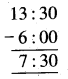Question 3.
From 5:00 am to 10:45 pm
Solution:
Time gap from 5:00 pm to 10:45 pm
Time gap from 5:00 pm to 10:00 pm = 5 hours
Time gap from 10:00 pm to 10:45 pm = 45 minutes
Hence, time gap from 5:00 to 10:45 pm = 5 hours 45 minutes

Question 4.
From 9:00 am to 2:30 am (next morning)
Solution:
Time gap from 9:00 pm to 2:30 am (next morning)
Time gap from 9:00 pm to 12:00 mid night = 3 hours
Time gap from 12:00 mid night to 2:00 am = 2 hours
Time gap from 2:00 am to 2:30 am = 30 minutes
Hence, time gap from 9:00 pm to 2:30 am (next morning) = 5 hours 30 minutesQuestion 4.
A bank opens at 9:30 am and closes at 5:00 pm. How many working hours are there ?
Solution:
Time gap from 9:30 am to 10:00 am
= 30 minutes Time gap from 10:00 am to 12:00 noon = 2 hours
Time gap from 12:00 noon to 5:00 pm = 5 hours
Hence, time gap from 9:00 am to 5:00 pm = 7 hours 30 minutes
Therefore, working hours of the bank = 7 hours 30 minutes

Question 5.
A bus starts from Chandigarh at 7:30 am and reaches Shimla at 10:50 am. How much time is taken by the bus to reach Shimla ?
Solution:
Time gap from 7:30 am to 8:00 am = 30 minutes
Time gap from 8:00 am to 10:00 am = 2 hours
Time gap from 10:00 am to 10:50 am = 50 minutes
Time gap from 7:30 am to 10:50 am
= 30 minutes + 2 hours + 50 minutes
= 2 hours + 80 minutes
= 2 hours + 60 minutes + 20 minutes
= 2 hours + 1 hour + 20 minutes
= 3 hours 20 minutes
Therefore, time taken by the bus to reach Shimla = 3 hours 20 minutes

Second Method :
The time at which the bus reaches Shimla = 10:50 am
The time at which the bus starts from Chandigarh = 7:30 am
= 3:20 hours
The time taken by bus to reach Shimla = 3 hours 20 min.Question 6.
A boy goes to school at 7:30 am and returns back from school at 2:45 pm. How much time does he spend in the school ?
Solution:
The time when the boy goes to school = 7:30 am
The time when the boy returns back = 2.45 am
The time gap from 7:30 am to 8:00 am = 30 minutes
The time gap from 8:00 am to 12:00 noon = 4 hours
The time gap from 12:00 noon to 2:00 pm = 2 hours
The time gap from 2:00 pm to 2:45 pm = 45 minutes
Total time
= 30 minutes + 6 hours + 45 minutes
= 6 hours + 75 minutes = 6 hours + 60 minutes + 15 minutes
= 6 hours + 1 hour + 15 minutes
= 7 hours 15 minutes
Therefore, time spent in the school = 7 hours 15 minutes

Second Method :
2:45 pm = 2:45 + 12:00 = 14:45 o’clock
The time when the boy returns back = 14:45 o’clock
The time when the boy goes to school = 7:30 o’clock
The time spent in the school by boy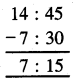= 7 hours 15 minutes